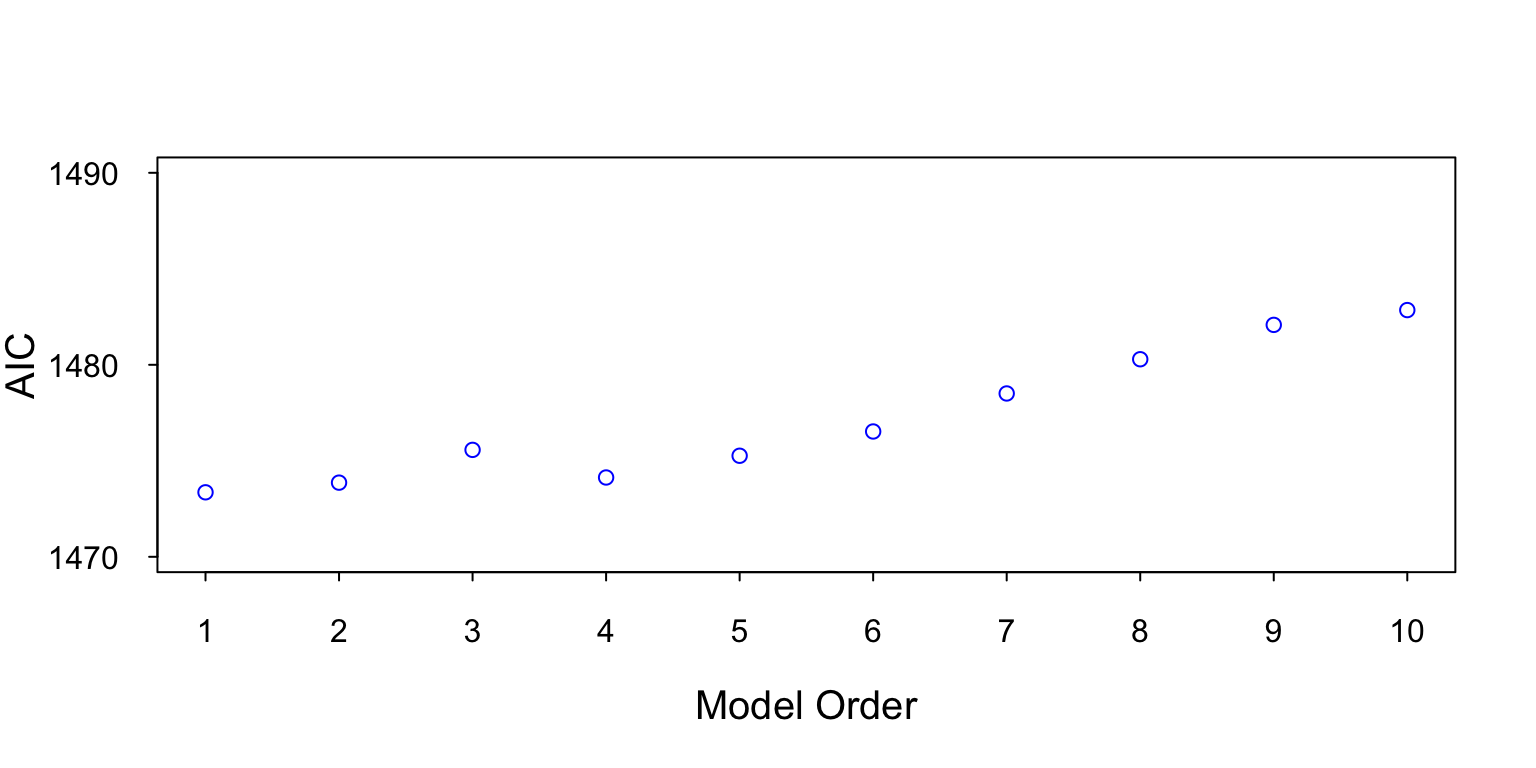## 1 Model Order Selection

This section provides a brief introduction to autoregressive (AR) model order estimation. An AR model of order p (AR[p]) can model up to p peaks in a PSD. So, if we wanted to estimate a PSD with 4 peaks we would use an AR model. Unfortunately, we don’t always know the number of peaks. Note, however we choose the model order we don’t want to over fit the model. Meaning we want to choose the minimum model order that can model the important features. Over fitting the model requires more parameters and adds bias and variance to our estimate.

Model order estimators are mathematical tools that let you estimate the order of the process that produced the data. The Akaike Information Criterion (AIC) is a model order estimator built into the `arima` function. The AIC calculates how well model fits the data (lower is better).

The `arima` function inputs the model order and the data. The function produces a number of outputs, but we only use the AIC. Using the `arima` function with `\$aic` outputs the AIC associated with the input model order. Below is an example of using the `arima` function to find the model order.

## 2 Example

In this example we use data from an AR model and then use the AIC to estimate the order of the AR model that produced the data. Note the example is implemented in the R programing language. First, we create data using an AR model.

``````set.seed(1)
N <- 512
y_ar1 <- rep(0,N)
x <- rnorm(N)
a <- 0.9

for (n in 2:N)
y_ar1[n] <- -a*y_ar1[n-1] + x[n] ``````

Next, we use the data to estimate the model order. We calculate the AIC for AR models AR to AR. The model with the lowest AIC is the model that fits the data. If two models have similar AICs we should use the lower order model.

``````aicAR <- rep(0,10)
for (modelOrder in 1:10) {
aicAR[modelOrder] <- arima(y_ar1, order = c(AR=modelOrder, 0, MA=0))\$aic
}``````

Figure 1 show the AIC value for AR model orders from 1 to 10.Figure 1: This figure contains a plot of the AIC for model orders 1 to 10. The lowest AIC value is from the AR model, which is the true model order. The AIC is not perfect and sometimes will not get the exact right answer.

Note a similar method could be use to find the model order of moving average (MA) and autoregressive moving avarage (ARMA) models.